# SBI PO Reasoning Questions 2019 (Day-09) High Level-New Pattern

SBI PO 2019 Notification is about to come and it is the most awaited exam among the aspirants. We all know that new pattern questions are introducing every year in the SBI PO exam. Further, the questions are getting tougher and beyond the level of the candidate’s expectations.

Our IBPS Guide is providing High-Level New Pattern Reasoning Ability Questions for SBI PO 2019 so the aspirants can practice it on a daily basis. These questions are framed by our skilled experts after understanding your needs thoroughly. Aspirants can practice these high-level questions daily to familiarize with the exact exam pattern. We wish that your rigorous preparation leads you to a successful target of becoming SBI PO.

[WpProQuiz 4894]

### Click Here for SBI PO Pre 2019 High-Quality Mocks Exactly on SBI Standard

Click Here to View Reasoning Questions in Hindi

(Directions 1–2): Study the following information carefully and answer the questions given below it.

There is a 5×5 matrix which can produce signals which in turn help in illumination of some bulbs. The rows of the matrix are denoted by @, %, &, £ and ¥ from bottom to top in the same order and the columns are denoted by A, B, C, D and E from left to right:

¥ row contains numbers which are consecutive multiple of ‘9’ starting from ‘36’.

£ row contains numbers which are consecutive multiple of ‘13’.

& row contains numbers which are consecutive multiple of ‘8’ starting from ‘32’.

% row contains numbers which are consecutive multiple of ‘7’.

@ row contains numbers which are consecutive multiple of ‘11’.

Note: If the starting number of a multiple is not given; then start the row from the same number.

E.g.: If a row contains numbers which are consecutive multiple of ‘5’. Then the row might be as follows;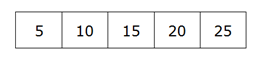The matrix helps in producing signals which is a string of numbers. There are four bulbs P, Q, R and S. Based on the outcome of the string one of the bulb blinks.

Condition for blink:-

I. If outcome of the string is below 80, the bulb Q blinks.

II. If outcome of the string is between 90 and 110, the bulb S blinks.

III. If outcome of the string is between 125 and 150, the bulb P blinks.

IV. If outcome of the string is between 175 and 200, the bulb R blinks.

V. If none of the above condition follows then, no bulb blinks.

For outcome of the string:

I. If all the numbers of the string is an even number then, outcome is obtained by multiplying unit digit of all the two digit numbers.

II. If a prime number is followed by another prime number then, outcome is obtained by sum of all two digits number.

III. If a prime number is preceded by a perfect square then, outcome is obtained by multiplying tenth place of all the numbers.

1) If Y = £B &E £A @D, then which of the following bulb blinks?

a) R

b) P

c) Q

d) S

e) None blinks

2) If X = ¥C @D £B @B, then which of the following bulb blinks?

a) R

b) Q

c) P

d) S

e) None blinks

(Directions 3–5): Study the following information carefully and answer the questions given below it.

Twelve boxes marked A to L as in alphabetical order are placed in the form of a 3 x 4 matrix. The rows of the matrix are denoted as 1, 2 and 3 from top to bottom and the columns are denoted as #, &, % and @ from right to left in the same order. The blocks contains twelve different articles viz. Pen, Watch, LED, Toy, Bat, Book, Ring, Banana, Cheery, Ball, Rose and Mango. All the information is not necessary in same order.

Note: When a box is said to be ‘beside /adjacent /between or at a gap of’ it can be either horizontally or vertically.

The box having Rose is kept second to left of box L. The box having Ring is kept just below box L. Box J is kept besides the box having Ring. The box having Mango is kept exactly between the box C and the box B. Box D is kept second to left of box E.

The box J has five neighboring boxes. Box having Banana is kept adjacent to box B. The box having Banana is kept exactly below box D. Box I is kept at a gap of one from box G. Box H is kept adjacent to box A.

Box J having Pen is kept exactly between box having Ball and box C. Box B neither contains Ball nor Rose. The box having Bat is kept adjacent to box H, which neither contains Mango nor kept adjacent to box having Ball.

The box having LED is kept exactly between box having Toy and box B. The box having Watch is kept at a gap of one from box G. Three boxes are neighbors of box I. Box K is kept exactly between the box having Book and box A.

3) Which of the following position correctly represent the box having Rose?

a) 1%

b) 2&

d) 3%

e) None of these

4) Which of the following box contains LED?

a) L

b) F

c) A

d) G

e) None of these

5) Which of the following statements is/are true?

I. Box G contains Mango is kept immediate left of the one which contains Cheery.

II. The box having Pen is kept second to left of the box which contain Watch.

III. The box which contains Book and the box having Banana are kept together.

IV. The box having Ball is kept exactly between box H and the one contains Pen.

a) Only I and IV

b) Only III

c) Only III and IV

d) Only II and IV

e) None of these

Directions (6 -8): Study the following information to answer the given questions.

There are two rows given. To find out the resultant of a particular row we need to follow the following condition:-

Condition 1: If an even number is followed by an odd number (prime number) then the resultant will be the addition of both the numbers.

Condition 2: If an odd number (prime number) is followed by a perfect square then the resultant will be the difference between the numbers.

Condition 3: If an odd number (prime number) is followed by another odd number (non-prime) then the resultant will be the product of both the numbers.

Condition 4: If an odd number (non-prime) is followed by an even number then the resultant will be the product of the numbers.

Condition 5: If an odd number (non-prime number) is followed by a cube number then the resultant will be the addition of both the numbers.

Condition 6: If an even number is followed by a perfect square then the resultant will be the product of both the numbers.

Note: Give preference to Condition (5) when both Condition (4) and (5) follows.

6) If the resultant of row 2 is 362 more than the resultant of row 1. Then find the value of X?

13        64     X

17    21    64

a) 13

b) 8

c) 16

d) 9

e) None of the above

7) Which of the following is the difference of the resultant of first and second row?

32        49     73

15    27    36

a) 131

b) 127

c) 141

d) 124

e) None of the above

8) If X is 1/5th the value of resultant of the first row then what will be the resultant of the second row?

28     9    53

21    36    X

a) 915

b) 817

c) 493

d) 978

e) None of these

(Directions 9–10): The given questions are based on five, 4-letter words which are given below. Study the following information carefully and answer the questions given below it.

CREW RISK MART COLD FARM

9) If all the letters within given words are arranged in reverse alphabetical order and thus newly formed words are rearranged as they appear in dictionary then, which of the following word appears third?

a) RISK

b) FARM

c) CREW

d) COLD

e) MART

10) If all the letters within given words are written together in an alphabetical order without any space then, how many letters are there in alphabetical series between sixth letter from left end and fifth letter from right end?

a) 7

b) 11

c) 5

d) 15

e) 9

Answers:

(Directions 1–2):

We have:

• ¥ row contains numbers which are consecutive multiple of ‘9’ starting from ‘36’.
• £ row contains numbers which are consecutive multiple of ‘13’.
• & row contains numbers which are consecutive multiple of ‘8’ starting from ‘32’.
• % row contains numbers which are consecutive multiple of ‘7’.
• @ row contains numbers which are consecutive multiple of ‘11’.

From above given statements we have: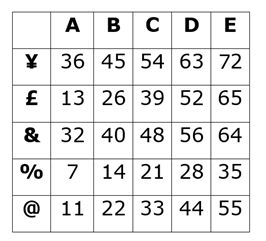1) Answer: C

We have:

Y = £B &E £A @D

After placing value of strings from given matrix, we have:

Y = 26 64 13 44

Clearly, a prime number is preceded by a perfect square then, outcome of the string is product of digits at tenth place.

Thus, Y = (2 x 6 x 1 x 4) = 48

Clearly, condition I follows. Thus, bulb ‘Q’ blinks.

Hence, option C is correct choice.

2) Answer: A

We have:

X = ¥C @D £B @B

After placing value of strings from matrix, we have:

X = 54 44 26 22

Clearly, all the numbers of string are even numbers.

Then, outcome is the product of digits at one’s place.

Thus, X = (4 x 4 x 6 x 2) = 192

Clearly, condition IV follows, thus bulb R blinks.

Hence, option A is correct choice.

(Directions 3–5):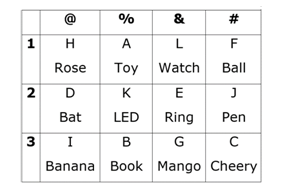We have:

• The box having Rose is kept second to left of box L.
• The box having Ring is kept just below box L, that means we have four possible place for box L, in case (1) box L is kept at 1&, in case (2) L is kept at 1#, in case (3) L is kept at 2& and in case (4) L is kept at 2#.
• Box J is kept besides the box having Ring.
• The box J has five neighboring boxes, that means case (2) is not valid, in case (1) J is kept at 2#, in case (3) J is kept at 3%, in case (4) J is kept at 3&.
• Box J having Pen is kept exactly between box having Ball and box C, that means we have four possible place for box C, in case (1a) box C is kept at 3#, in case (1b) box C is kept at 1#, in case (3) box C is kept at 3&, in case (4) box C is kept at 3#.
• The box having Mango is kept exactly between the box C and the box B. Box B neither contains Ball nor Rose, that means in case (1a) box B is kept at 3%, in case (1b) 1&, in case (3) box B is kept at 1&, in case (4) box B is kept at 1#.

Based on above given information we have: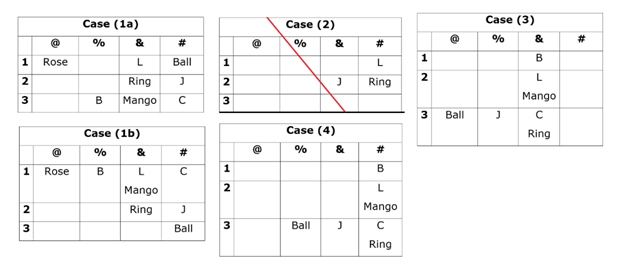Case (2) is not valid as The box J has five neighboring boxes.

Again, we have:

• The box having LED is kept exactly between box having Toy and box B.
• Box having Banana is kept adjacent to box B, that means case (1b) & case (4) are not valid, in case (1a) the box having banana is kept at [email protected], in case (3) the box having banana is kept at 1#.
• The box having Banana is kept exactly below box D, that means case (3) is not valid and in case (1a) box D is kept at [email protected]
• Box D is kept second to left of box E, that means box E is kept at 2&.

Based on above given information we have: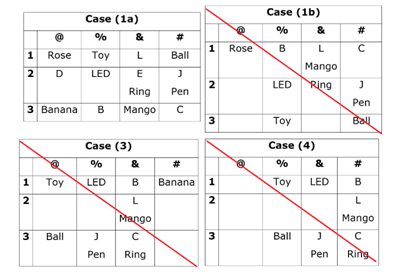Case (1b) & case (4) as box having Banana is kept adjacent to box B and case (3) is not valid as the box having Banana is kept exactly below box D.

Again, we have:

• Three boxes are kept neighbor of box I, which neither contains Ball nor Rose, that means box I contains Banana.
• Box I is kept at a gap of one from box G, that means we have two possible place for box G, in case (1a) box G is kept at [email protected], in case (1c) box G is kept at 3&.
• The box having Watch is kept at a gap of one from box G, that means in case (1a) the box having Watch is kept at 1&, in case (1c) the box having Watch is kept at 3&.
• The box having Bat is kept adjacent to box H, which neither contains Mango nor kept adjacent to box having Ball.
• Box H is kept adjacent to box A.
• Box K is kept exactly between the box having Book and box A, that means in case (1a) box D contains Bat and box H contains LED, thus case (1a) is not valid, in case (1c) box D contains Bat and box H contains Rose.

Based on above given information we have final arrangement as follow: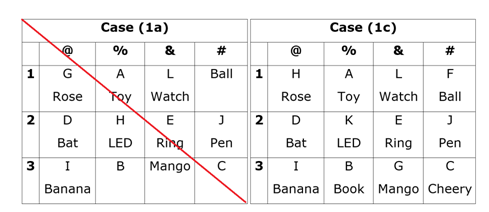Case (1a) is not valid as Box K is kept exactly between the box having Book and box A.

3) Answer: C

Clearly, Box A having Rose is placed at ‘[email protected]’.

Hence, option C is correct choice.

4) Answer: E

Clearly, box K contains LED.

Hence, option E is correct choice.

5) Answer: E

Clearly, only statement I and III are true.

Hence, option E is correct choice.

Directions (6 -8):

6) Answer: B

We have:

13        64     X

17    21    64

For 2nd row:

• An odd number (prime number) is followed by odd number (non-prime number) so, 17 x 21 = 357.
• Now, the row is 357 64, an odd number (non-prime number) followed by a cube number so, 357 + 64 = 421.
• As, resultant of difference of both rows = 362

Thus, resultant of row 1 = (421 – 362) = 59.

For 1st row:

• An odd number (prime number) is followed by another odd number (non-prime number) so, 64 – 13 = 51.
• Now, the row is 51 X, odd number (non-prime number) followed by X to get resultant 59.

Thus, 51 + 8 = 59, this follows condition 5.

Thus, required value of X = 8.

Hence, option B is correct choice.

7) Answer: E

We have:

32    49     73

15    27     36

For 1st row:

• An even number followed by perfect square, so 32 x 49 = 1568.
• Now, the row is 1568 73, an even number followed by odd number (prime number) so, 1568 + 73 = 1641.

Thus, resultant of row 1 = 1641.

For 2nd row:

• An odd number (non-prime number) followed by a cube number so, 15 + 27 = 42.
• Now, the row is 42 36, an even number is followed by a perfect square so, 42 x 36 = 1512.

Thus, resultant of row 2 = 1512.

Thus, required difference = (1641 – 1512) = 129.

Hence, option E is correct choice.

8) Answer: B

We have:

28     9    53

21    36    X

For 1st row:

• An even number followed by perfect square so, 28 x 9 = 252.
• Now, the row is 252 53, an even number followed by an odd number (prime number) so, 252 + 53 = 305.

Thus, resultant of 1st row = 305.

For 2nd row:

• Clearly, value of X = 305/5 = 61.
• An odd number (non-prime number) followed by an even number, so 21 x 36 = 756.
• An even number followed by an odd number (prime number) so, 756 + 61 = 817.

Thus, resultant of 2nd row = 817.

Hence, option B is correct choice.

(Directions 9–10):

9) Answer: A

We have:

CREW RISK MART COLD FARM

After rearranging all the letters within words, we get:

WREC SRKI TRMA OLDC RMFA

Thus, “SRKI” appears third in dictionary.

Hence, option A is correct choice.

10) Answer: A

We have:

CREW RISK MART COLD FARM

After rearranging all the letters in alphabetical order we get:

AACCDEFIKLMMORRRRSTW

Seventh letter from left end -> F

Sixth letter from right end -> R

Thus, number of letters between ‘F’ and ‘R’ in an alphabetical series = 11.

Hence, option B is correct choice.

### Click Here for SBI PO Pre 2019 High-Quality Mocks Exactly on SBI Standard

Daily Practice Test Schedule | Good Luck

 Topic Daily Publishing Time Daily News Papers & Editorials 8.00 AM Current Affairs Quiz 9.00 AM Current Affairs Quiz (Hindi) 9.30 AM NIACL AO Prelims – Reasoning 10.00 AM NIACL AO Prelims – Reasoning (Hindi) 10.30 AM NIACL AO Prelims – Quantitative Aptitude 11.00 AM NIACL AO Prelims – Quantitative Aptitude (Hindi) 11.30 AM Vocabulary (Based on The Hindu) 12.00 PM NIACL AO Prelims – English Language 1.00 PM SSC Practice Questions (Reasoning/Quantitative aptitude) 2.00 PM IBPS Clerk – GK Questions 3.00 PM SSC Practice Questions (English/General Knowledge) 4.00 PM Daily Current Affairs Updates 5.00 PM SBI PO/IBPS Clerk Mains – Reasoning 6.00 PM SBI PO/IBPS Clerk Mains – Quantitative Aptitude 7.00 PM SBI PO/IBPS Clerk Mains – English Language 8.00 PM
0 0 votes
Rating0 Comments
Inline Feedbacks
View all comments